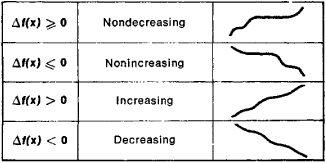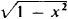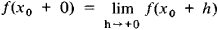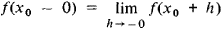# Monotonic Function

(redirected from Nonincreasing function)

## monotonic function

[¦män·ə¦tän·ik ′fəŋk·shən]
(mathematics)

## Monotonic Function

(or monotone function), a function whose increments Δf(x) = f(x′) − f(x) do not change sign when Δx = x′ − x > 0; that is, the increments are either always nonnegative or always nonpositive. Somewhat inaccurately, a monotonic function can be defined as a function that always varies in the same direction. Different types of monotonic functions are represented in Figure 1. For example, the function y = x3 is an increasing function. If a function f(x) has a derivative f′(x) that is nonnegative at every point and that vanishes only at a finite number of individual points, then f(x) is an increasing function. Similarly, if f′(x) ≤ 0 and vanishes only at a finite number of points, then f(x) is a decreasing function.Figure 1

A monotonicity condition can hold either for all x or for x on a given interval. In the latter case, the function is said to be monotonic on this interval. For example, the function y =increases on the interval [−1,0] and decreases on the interval [0, +1]. A monotonic function is one of the simplest classes of functions and is continually encountered in mathematical analysis and the theory of functions. If f(x) is a monotonic function, then the following limits exist for any X0:andReferences in periodicals archive ?
It is clear that if [phi] is a nondecreasing function or a nonincreasing function, then [phi] is an MT-function.
Similarly, [mathematical expression not reproducible] is a monotonous and nonincreasing function of [c.sub.r].
The quantized satisfaction with given value of QoS metrics can be described by the utility function [U.sub.j](x), a nonincreasing function with respect to the give metrics [l.sub.j], j = 1, ..., J.
Let (u, v) be a solution of problem (1)-(5); then E(t) is a nonincreasing function for t [greater than or equal to] 0; that is,
Because [[??].sub.4] [less than or equal to] 0, it can be concluded that [V.sub.4](t) is a nonincreasing function; then [V.sub.4](s(0), [[??].sub.2](0)) is bounded.
Let b be a nonincreasing function from [0, [infinity]) into (0, [infinity]) with [[integral].sup.[infinity].sub.0] b(t)dt = [infinity]; and let [TAU] be a function from X into [0, [infinity]) with [TAU](y) [less than or equal to] [TAU](x) + p(x,y) for x, y [member of] X.
Hence, by a classical lemma of Hardy, if f is a nonnegative, nonincreasing function on (0, 1], then
where A(w) is the set of random wealths [X.sub.T] that can be generated at maturity T > 0 with an initial wealth w, and where [A.sub.[xi]] (w) is the subset of random wealths that are almost surely anticomonotonic with [[xi].sub.T] (in other words, that are almost surely a nonincreasing function of [[xi].sub.T]).
It is noted that the frequency of the harmonic potential is [omega] = [square root of (2[[OMEGA].sub.1](1 + 2[[OMEGA].sub.1][t.sup.2]))], and the coefficients of two- and three-body interactions are [MATHEMATICAL EXPRESSION NOT REPRODUCIBLE IN ASCII], which are monotone nonincreasing function of time t.
This procedure exploits the fact that, by definition, [[??].sup.0] is a nonincreasing function of [v.sub.min].
Thus V (t) is a nonincreasing function of time; that is,
([H.sub.4]) There exists a nonincreasing function [[psi].sub.2] [member of] C (R, (0, +[infinity])) with [[psi].sub.2](s) [less than or equal to] 1 for s [member of] R and such that

Site: Follow: Share:
Open / Close